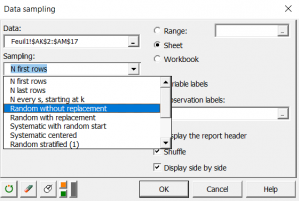# Data sampling

Use this tool to generate a subsample of observations from a set of univariate or multivariate data. Available in Excel using the XLSTAT add-on statistical software.## Use of data sampling

Sampling is one of the fundamental data analysis and statistical techniques. Sampling aims at extracting a sample of size n from a dataset.

Samples are generated to:

1. Test an hypothesis on one sample, then test it on another;
2. Obtain very small tables which have the properties of the original table.

To meet these different situations, several methods have been proposed.

## XLSTAT data sampling options

XLSTAT offers the following methods for generating a sample of N observations from a table of M rows:

• N first rows: The sample obtained is taken from the first N rows of the initial table.
• N last rows: The sample obtained is taken from the last N rows of the initial table. This method is only used if it is certain that the values have not been sorted according to a particular criterion which could introduce bias into the analysis
• N every s starting at k: The sample is built extracting N rows, every s rows, starting at row k
• Random without replacement: Observations are chosen at random and may occur only once in the sample
• Random with replacement: Observations are chosen at random and may occur several times in the sample
• Systematic from random start: From the j'th observation in the initial table, an observation is extracted every k observations to be used in the sample. j is chosen at random from among a number of possibilities depending on the size of the initial table and the size of the final sample. k is determined such that the observations extracted are as spaced out as possible
• Systematic centered: Observations are chosen systematically in the centers of N sequences of observations of length k
• Random stratified (1): Rows are chosen at random within N sequences of observations of equal length, where N is determined by dividing the number of observations by the requested sample size;
• Random stratified (2): Rows are chosen at random within N strata defined by the user. In each stratum, the number of sampled observations is proportional to the relative frequency of the stratum.
• Random stratified (3 ): Rows are chosen at random within N strata defined by the user. In each stratum, the number of sampled observations is proportional to a relative frequency supplied by the user.
• User defined: A variable indicates the frequency of each observation within the output sample.
• Training and test sets: Data are split into two parts – a training set and a test set. The rows of each set are randomly drawn from the initial dataset. The size of the training set is defined by a number of rows.
• Training and test sets (%): Data are split into two parts – a training set and a test set. The rows of each set are randomly drawn from the initial dataset. The size of the training set is defined by a row number percentage from the initial data set.### analyze your data with xlstat

14-day free trial

Included in

Related features